Different Method to find Index numbers, Business Mathematics and Statistics

# Different Method to find Index numbers, Business Mathematics and Statistics - Business Mathematics and Statistics - B Com

Weighted Aggregate Method

In this method, appropriate weights are assigned to various commodities to reflect their relative importance in group. For the construction of price index number, quantity, weights are used i.e. amount of quantity consumed, purchased or marketed.
By using different systems of weighting we get a number of formulae which are as follows—

Laspeyres’ Price Index

In this method the base year quantities are taken as weights. Symbolically—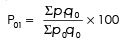The main advantage of this method is that it uses only base year quantity ; therefore there is no need to keep record of quantity consumed in each year.

Disadvantage It is a common knowledge that the consumption of commodity decreases with relative large increase in price and vice versa. Since in this method base year quantity is taken as weights, it does not take into account the change in consumption due to increase or decrease in prices and hence may give a biased result.

Paasche’s Method In this method current year quantities are taken as weights. Symbolically—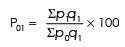Dorbish and Bowley’s Method This method is the simple arithmetic mean of the Laspeyres’ and Paasche’s indices. This index takes into account the influence of quantity weights of both base period and current period. The formula is as follows: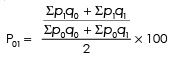Fisher ‘Ideal’ Method This method is the geometric mean of Laspeyres’ and Paasche’s indices. The formula is as follows—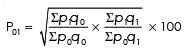Because of the following advantages this method is seldom referred as ideal method—
(1) The formula takes into account both base year and current year quantities as weights, and hence avoids bias associated with the Laspeyres’ and Paasche’s indices.
(2) The formula is based on geometric mean which is considered to be the best average for constructing index numbers.
(3) This method satisfies unit test, time reversal test and factor reversal test.

(1) This method is more time consuming than other methods.
(2) It also does not satisfy circular test

Marshall-Edgeworth Method In this method arithmetic mean of base year and current year quantities are taken as weights symbolically—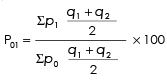Kelly’s Method
In this method fixed weights are taken as weights. This method is sometimes referred to as aggregative index with fixed weights method. Fixed weights are quantities which may be for some particular period (not necessarily of base year or the current year) and this is kept constant all the time. The formula is as follows—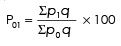(1) This index does not require yearly changes in the weights.

This method does not take into account the weight of either the base year or the current year.

Example 3 : Compute the price index as per the following methods :
(1) Laspeyres’
(2) Paasche’s
(3) Fisher
(4) Bowley’s
(5) Marshall - Edgeworths from the following :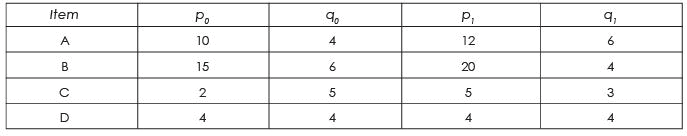Solution :
Table :
Calculation of various indices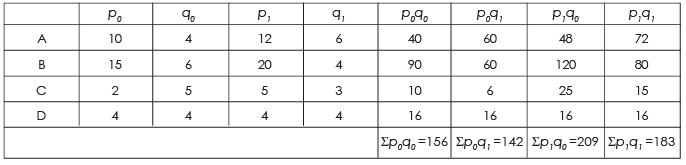(i) Laspeyres’ Index Number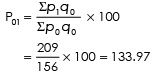(ii) Paasche’s Index Number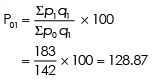(iii) Bowley’s Index Number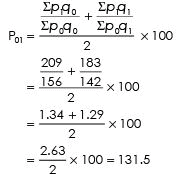(iv) Fisher’s Index Number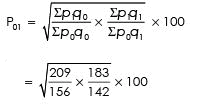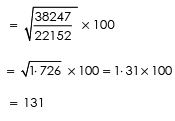(v) Marshall-Edgeworth Index Number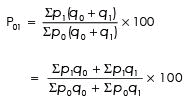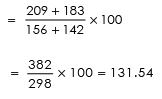The document Different Method to find Index numbers, Business Mathematics and Statistics | Business Mathematics and Statistics - B Com is a part of the B Com Course Business Mathematics and Statistics.
All you need of B Com at this link: B Com

115 videos|142 docs

## FAQs on Different Method to find Index numbers, Business Mathematics and Statistics - Business Mathematics and Statistics - B Com

 1. How are index numbers calculated?Ans. Index numbers are calculated by dividing the value of a particular variable in a given period by the value of the same variable in the base period, and then multiplying the result by 100. This calculation is done to measure the relative change in the variable over time.
 2. What is the purpose of using index numbers in business mathematics and statistics?Ans. The purpose of using index numbers in business mathematics and statistics is to analyze and compare changes in variables over time. Index numbers provide a standardized way to measure and compare the relative changes in variables such as prices, production levels, or economic indicators. They help in understanding trends, making forecasts, and making informed business decisions.
 3. Can index numbers be negative?Ans. Yes, index numbers can be negative. Negative index numbers indicate a decrease or decline in the value of the variable being measured compared to the base period. For example, if an index number for prices is -10, it means that prices have decreased by 10% compared to the base period.
 4. How can index numbers be used in financial analysis?Ans. Index numbers are commonly used in financial analysis to evaluate the performance of financial markets, stocks, or investment portfolios. By comparing the index numbers of different stocks or market indices, investors can assess the relative performance and volatility of different investments. Index numbers also help in tracking the overall financial market trends and making investment decisions based on market movements.
 5. What are the limitations of using index numbers?Ans. While index numbers are useful for measuring relative changes, they have certain limitations. One limitation is that index numbers can be influenced by outliers or extreme values in the data, which may distort the overall picture. Additionally, index numbers may not capture all relevant factors that can affect the variable being measured. It is important to interpret index numbers cautiously and consider additional factors before making conclusions or decisions based solely on index values.

115 videos|142 docsExplore Courses for B Com examSignup to see your scores go up within 7 days! Learn & Practice with 1000+ FREE Notes, Videos & Tests.
10M+ students study on EduRev
Track your progress, build streaks, highlight & save important lessons and more!
Related Searches

,

,

,

,

,

,

,

,

,

,

,

,

,

,

,

,

,

,

,

,

,

,

,

,

;